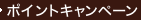•### Inverse Problems of Mathematical Physics

• ただいまウェブストアではご注文を受け付けておりません。 ⇒古書を探す
• 製本 Hardcover:ハードカバー版／ページ数 276 p.
• 言語 ENG
• 商品コード 9789067643962
• DDC分類 515

### Full Description

The theory of Inverse Problems of Mathematical Physics is a vast and intensively developing field of modern mathematics. This book deals mostly with the theoretical aspects and treats some applications, as well as numerical methods of solving problems. In the introductory chapter the concept of mathematical modelling is given, the general differences between direct and inverse problems are outlined and strict mathematical definitions of correct and ill-posed problems are given. The following chapters deal with: - Approximate methods of solution of ill-posed problems - Integral geometry problems - One dimensional inverse problems - Inverse problems for the coupled Maxwell and Lami systems - Numerical solution of inverse problems: some examples This books is intended to serve as an introduction for specialists in other fields, and thus emphasizes the basic general principles and approaches to the solution of various problems, whereby concrete examples of results obtained are given.